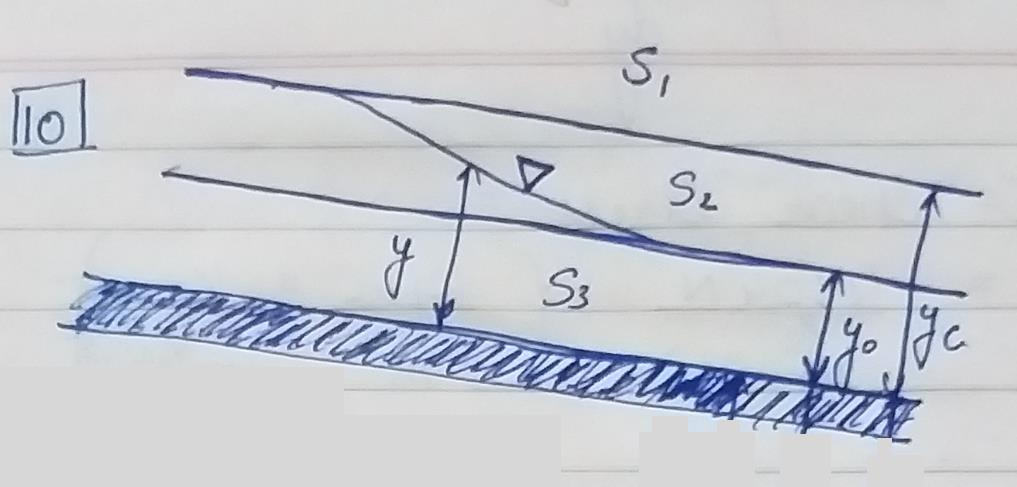Courses

# Test: Soil Mechanics & FM- 4

## 25 Questions MCQ Test Civil Engineering SSC JE (Technical) | Test: Soil Mechanics & FM- 4

Description
This mock test of Test: Soil Mechanics & FM- 4 for Civil Engineering (CE) helps you for every Civil Engineering (CE) entrance exam. This contains 25 Multiple Choice Questions for Civil Engineering (CE) Test: Soil Mechanics & FM- 4 (mcq) to study with solutions a complete question bank. The solved questions answers in this Test: Soil Mechanics & FM- 4 quiz give you a good mix of easy questions and tough questions. Civil Engineering (CE) students definitely take this Test: Soil Mechanics & FM- 4 exercise for a better result in the exam. You can find other Test: Soil Mechanics & FM- 4 extra questions, long questions & short questions for Civil Engineering (CE) on EduRev as well by searching above.
QUESTION: 1

Solution:
QUESTION: 2

Solution:
QUESTION: 3

### The loss of head at exit of a pipe is given by the formula hL =

Solution:
QUESTION: 4

Loss of energy at the entrance of a pipe from a large vessel is given by the formula

Solution:
QUESTION: 5

The stream function for a two dimensional flow is given by Y = 2xy. The velocity at (2,2) is

Solution:
QUESTION: 6

In case of S2 water surface profile (y = depth of flow, yo = normal depth and yc = critical depth)

Solution:QUESTION: 7

A surge tank is provided in the hydro power scheme to

Solution:
QUESTION: 8

If the Froude number characterizing flow in an open channel is less than unity an increase in channel width causes the water surface elevation to

Solution:
QUESTION: 9

In case of broad crested weire, the depth of water h at the end of sill is given by

Solution:
QUESTION: 10

The horizontal to vertical side slope in case of Cippoletti weir?

Solution:
QUESTION: 11

The concept of boundary layer was first introduced by

Solution:
QUESTION: 12

The thickness of boundary layer at the leading (entrance) edge will be

Solution:
QUESTION: 13

On a stationary thin plate, having flow in the direction of length of the patle, if discharge is reduced then the thickness of boundary layer will

Solution:
QUESTION: 14

Laminar sublayer is a thin boundary layer, formed just adjacent to the boundary in

Solution:
QUESTION: 15

As compared to laminar flow, in turbulent flow, boundary layers will be

Solution:
QUESTION: 16

The tangential force exerted by the shearing stresses on an object submerged in a fluid is known as

Solution:
QUESTION: 17

Drag and lift forces acting on an object placed is a fluid stream are caused by

Solution:
QUESTION: 18

The component of resultant fluid dynamic force,in the flow direction is called

Solution:
QUESTION: 19

Lift force is induced in a object, if

Solution:
QUESTION: 20

For a stream line body, the predominant drag force is

Solution:
QUESTION: 21

The tendency of a liquid surface to contract is due to the following property

Solution:
QUESTION: 22

The friction drag is caused by

Solution:
QUESTION: 23

When an ideal fluid flows past a sphere

Solution:
QUESTION: 24

With the same cross-sectional area and placed in the same turbulent flow, the largest drag will be experienced by

Solution:
QUESTION: 25

The friction drag is more than pressure drag for

Solution: